The OpenFOAM Foundation
polynomialSolidTransport< Thermo, PolySize > Class Template Reference

Transport package using polynomial functions for solid kappa. More...

Inheritance diagram for polynomialSolidTransport< Thermo, PolySize >: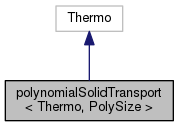[legend]
Collaboration diagram for polynomialSolidTransport< Thermo, PolySize >:[legend]

Public Member Functions

polynomialSolidTransport (const word &, const polynomialSolidTransport &)
Construct as named copy. More...

polynomialSolidTransport (const dictionary &dict)
Construct from dictionary. More...

autoPtr< polynomialSolidTransportclone () const
Construct and return a clone. More...

scalar mu (const scalar p, const scalar T) const
Dynamic viscosity [kg/ms]. More...

scalar kappa (const scalar p, const scalar T) const
Thermal conductivity [W/mK]. More...

vector Kappa (const scalar p, const scalar T) const
Thermal conductivity [W/mK]. More...

scalar alphah (const scalar p, const scalar T) const
Thermal diffusivity of enthalpy [kg/ms]. More...

void write (Ostream &os) const
Write to Ostream. More...

void operator= (const polynomialSolidTransport &)

void operator+= (const polynomialSolidTransport &)

void operator*= (const scalar)

Static Public Member Functions

static autoPtr< polynomialSolidTransportNew (const dictionary &dict)

static word typeName ()
Return the instantiated type name. More...

Static Public Attributes

static const bool isotropic = true
Is the thermal conductivity isotropic. More...

Friends

polynomialSolidTransport operator+ (const polynomialSolidTransport &, const polynomialSolidTransport &)

polynomialSolidTransport operator* (const scalar, const polynomialSolidTransport &)

Ostreamoperator (Ostream &, const polynomialSolidTransport &)

Detailed Description

template<class Thermo, int PolySize = 8> class Foam::polynomialSolidTransport< Thermo, PolySize >

Transport package using polynomial functions for solid kappa.

Usage
Property Description
kappaCoeffs<8> Thermal conductivity polynomial coefficients

Example of the specification of the transport properties:

transport
{
kappaCoeffs<8>     ( 1000 -0.05 0.003 0 0 0 0 0 );
}

The polynomial expression is evaluated as so: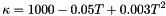Note
Thermal conductivity polynomial coefficients evaluate to an expression in [W/m/K].
Source files
Foam::Polynomial

Definition at line 81 of file polynomialSolidTransport.H.

◆ polynomialSolidTransport() [1/2]

 polynomialSolidTransport ( const word & name, const polynomialSolidTransport< Thermo, PolySize > & pt )
inline

Construct as named copy.

Definition at line 46 of file polynomialSolidTransportI.H.

◆ polynomialSolidTransport() [2/2]

 polynomialSolidTransport ( const dictionary & dict )

Construct from dictionary.

Definition at line 33 of file polynomialSolidTransport.C.

◆ clone()

 Foam::autoPtr< Foam::polynomialSolidTransport< Thermo, PolySize > > clone ( ) const
inline

Construct and return a clone.

Definition at line 58 of file polynomialSolidTransportI.H.

◆ New()

 Foam::autoPtr< Foam::polynomialSolidTransport< Thermo, PolySize > > New ( const dictionary & dict )
inlinestatic

Definition at line 69 of file polynomialSolidTransportI.H.

References dict, and polynomialSolidTransport< Thermo, PolySize >::mu().

Here is the call graph for this function: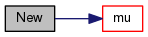◆ typeName()

 static word typeName ( )
inlinestatic

Return the instantiated type name.

Definition at line 158 of file polynomialSolidTransport.H.

◆ mu()

 Foam::scalar mu ( const scalar p, const scalar T ) const
inline

Dynamic viscosity [kg/ms].

Definition at line 82 of file polynomialSolidTransportI.H.

Referenced by polynomialSolidTransport< Thermo, PolySize >::New().

Here is the call graph for this function: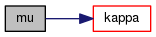Here is the caller graph for this function: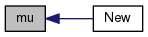◆ kappa()

 Foam::scalar kappa ( const scalar p, const scalar T ) const
inline

Thermal conductivity [W/mK].

Definition at line 94 of file polynomialSolidTransportI.H.

Here is the call graph for this function: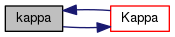Here is the caller graph for this function: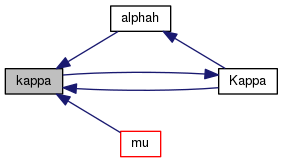◆ Kappa()

 Foam::vector Kappa ( const scalar p, const scalar T ) const
inline

Thermal conductivity [W/mK].

Definition at line 105 of file polynomialSolidTransportI.H.

Referenced by polynomialSolidTransport< Thermo, PolySize >::kappa().

Here is the call graph for this function:Here is the caller graph for this function: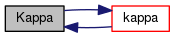◆ alphah()

 Foam::scalar alphah ( const scalar p, const scalar T ) const
inline

Thermal diffusivity of enthalpy [kg/ms].

Definition at line 117 of file polynomialSolidTransportI.H.

Referenced by polynomialSolidTransport< Thermo, PolySize >::Kappa().

Here is the call graph for this function: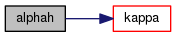Here is the caller graph for this function: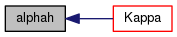◆ write()

 void write ( Ostream & os ) const

Write to Ostream.

Definition at line 52 of file polynomialSolidTransport.C.

Here is the call graph for this function: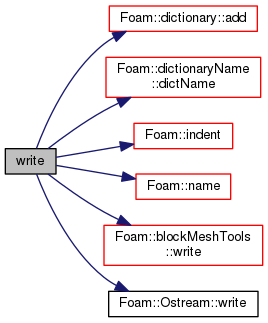◆ operator=()

 void operator= ( const polynomialSolidTransport< Thermo, PolySize > & )
inline

Definition at line 129 of file polynomialSolidTransportI.H.

◆ operator+=()

 void operator+= ( const polynomialSolidTransport< Thermo, PolySize > & )
inline

Definition at line 141 of file polynomialSolidTransportI.H.

References Y, Y1, and Y2.

◆ operator*=()

 void operator*= ( const scalar s )
inline

Definition at line 158 of file polynomialSolidTransportI.H.

References s(), Y1, and Y2.

Here is the call graph for this function: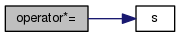◆ operator+

 polynomialSolidTransport operator+ ( const polynomialSolidTransport< Thermo, PolySize > & , const polynomialSolidTransport< Thermo, PolySize > & )
friend

◆ operator*

 polynomialSolidTransport operator* ( const scalar , const polynomialSolidTransport< Thermo, PolySize > & )
friend

◆ operator

 Ostream& operator ( Ostream & , const polynomialSolidTransport< Thermo, PolySize > & )
friend

◆ isotropic

 const bool isotropic = true
static

Is the thermal conductivity isotropic.

Definition at line 164 of file polynomialSolidTransport.H.

The documentation for this class was generated from the following files: